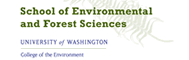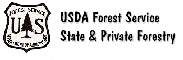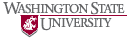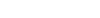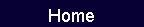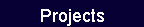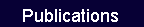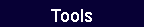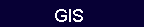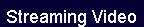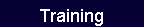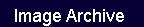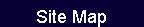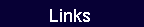# Chapter 9.  Energy

### Heating Values of Convetional Fuels

 Chapter9.  Energy

Proximate and Ultimate Analysis of Wood

Wood is usually converted into energy by burning. Combustion commences by evaporating the water present in the wood structure. Then combustible and noncombustible components are driven off at temperatures from 100˚ to 600˚ C. Table 9-1 gives the proximate analysis of wood and bark, showingthat about 75 to 85% of the wood can be volatilized. Carbon is oxidized in the final stage of combustion.

Combustion of wood involves two reactions:
(1) combining carbon from the wood with oxygen to form carbon dioxide and (2) combining hydrogen from the wood with oxygen to form water.

Table 9-1 also gives the  chemical analysis (termed ultimate analysis) of wood and bark, showing average percentage composition of carbon, hydro­gen, and oxygen. Neither ultimate nor proximate analyses vary greatly between species.

Part of the oxygen in these reactions comes from the oxygen already present in the wood, and the rest that is needed comes from the air. The minimum total amount of oxygen and hence air needed from these two sources can be theoretically calculated, but in practice more air than the theoretical amount is admitted to ensure complete combustion. This additional air is called excess air and the amount is carefully controlled in modern combustion systems.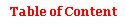Higher Heating Value (HHV)

Higher heating value (HHV) is a laboratory measurement of the stored chemical energy in a fuel and is expressed in British thermal units per pound (Btu/lb), joules per kilogram (J/kg), or megajoules per kilogram (MJ/kg). Calculations in this chapter are in Imperial units. See Appendix 1 for conver­sions between energy measures.

Table 9-2 presents average higher heating values for northwestern species. Wilson et al. (1987) have provided an extensive compilation of ultimate and proximate analysis and higher heating values for northwestern species. Note that HHV is measured for oven-dry wood (zero percent moisture content).

Differences between species, between wood and bark, and variations within a species reflect differ­ences in chemical composition, especially extrac­tives. In engineering calculations, it is common to use an average HHV of 9,000 Btu/dry lb for the more resinous conifers and 8,300 Btu/dry lb for the less resinous hardwoods.

Gross Heating Value (GHV)

Since a pound of fuelwood is generally not received in the oven-dry condition, HHV does not correctly represent the available potential heat. For example, a pound of wood fuel may contain 0.6 pound of oven-dry wood and 0.4 pound of water. In the wood fuel literature, moisture content is usually reported on a wet basis (Chapter 1), hence the moisture content (MCw) in this example is 40%. Since HHV assumes that this pound is entirely oven-dry wood, HHV must be reduced to reflect the actual amount of oven-dry wood present (Wd). The following equation represents the wood and water components in percent form:

Wd + MCw = 100.

Therefore, the gross heating value of a pound of wet wood is obtained by adjusting HHV for the wood moisture content as follows:

GHV  =  HHV (1 - MCw/100).

Thus GHV represents the available potential heat at a given moisture content and is equal to HHV only
if the wood is oven-dry. Continuing the example, if this wood has HHV of 9,000 Btu/lb, then

GHV  =  9,000 (1 - 40/100)  =  5,400 Btu/lb of oven-dry wood in the fuel.

Table 9-1.  Typical proximate and ultimate analysis of wood.

 Proximate (%) Ultimate (%) Volatile matter Fixed carbon Ash C H O N Ash SOFTWOODS Wood 77.2 22.0 1.6 52.9 6.3 39.7 0.1 1.0 Bark 73.3 23.7 3.0 53.1 5.9 37.9 0.2 2.3 HARDWOODS Wood 77.3 19.4 3.4 50.8 6.4 41.8 0.4 0.9 Bark 76.7 18.6 4.6 51.2 6.0 37.9 0.4 5.2

Source:  Arola  (1976).                                                 Note:  Ash percentages may differ since different data sources were averaged.

Table 9-2.  Higher heating value of wood and bark of some northwestern species (Btu/oven-dry lb).

 Species Wood Bark SOFTWOODS Douglas-fir 8,900 9,900 Lodgepole pine 8,600 10,100 Ponderosa pine 9,100 9,600 Redwood 9,200 - Sitka spruce 8,100 - True firs 8,300 9,100 Western hemlock 8,400 9,300 Western redcedar 9,700 8,700 White pine 9,600 - HARDWOODS Bigleaf maple 8,400 - Black cottonwood 8,800 9,000 Oregon ash 8,200 - Oregon white oak 8,100 - Red alder 8,000 8,600

Note:  Values are averaged from multiple sources and rounded to the nearest 100 Btu/lb. For additional details on these and other species, see Ince (1979)  and Wilson et al. (1987).Energy Losses

GHV does not represent the amount of heat available for use because it includes all the heat that can be extracted from the products of combustion, and there are several sources of loss that must be accounted for in determining the recoverable heat. Recoverable heat (RH) is important in comparing costs of fuels and fuel systems and represents the heat energy that, for example, produces steam for industrial purposes. The literature on these losses can sometimes be confusing because authors differ on which losses to consider and how some of them are calculated.

Losses due to the moisture content of wood are often referred to as the stack heat loss. A pound of wet fuel has a certain fraction that is oven-dry wood plus another fraction that is its water content. In the example above, there was 0.6 pound of oven-dry wood (Wd) and 0.4 pound of water (MCw = 40%). Formulas for eight forms of heat loss, numbered H1 through H8, are discussed below (Bethel 1977; Ince 1979).

H1.  Heat used to raise the temperature of water in the wood to the boiling point. In Btus this is

H1  =  (212 - T1)  *  MCw / 100

where T1  =  ambient temperature.

H2.  Heat required to vaporize the water in the wood.
In Btus this is

H2  =  970  *  MCw / 100.

H3.  Heat required to separate the bound water (water below fiber saturation point) from the cell walls.
In Btus this is

H3  =  136  *   MCb / 100

where MCb  =  fiber saturation point (see Chapter 1) if MCw  ≥  fsp

or MCb   =  MCw if MCw < fsp.

Usually it is assumed that  fsp = 30% MCod or 23% MCw.

H4.  Heat required to raise the temperature of the vaporized water to the temperature of the  exhaust gases. In Btus this is

H4  =  0.46  (T2 - 212)  *  MCw / 100

where  T2 = temperature of exhaust gases.

The average specific heat of water over the range from boiling point to exhaust temperature is about 0.46.

Losses H1, H2, and H4 are often combined and simplified into a single equation (Ince 1979):

MCw / 100  *  [970 + (212 - T1)  +  0.46  (T2 - 212)].

H5.  Heat required to evaporate water that forms when the  hydrogen component of wood is combusted.

Since wood is  approximately 6% hydrogen (Table 9-1), a pound of dry wood contains about 0.06 pound of hydrogen. During combustion, one of the chemical reactions is

2H2 + O2 ®2 H2O + 61,100  Btu/lb of H2.

By weight, water is one part hydrogen and eight parts oxygen. Therefore, 0.06 pound of hydrogen combines with 0.48 pound of oxygen to yield 0.54 pound of water, which also escapes via the stack gases. This water incurs losses involved with raising its temperature to the boiling point, evaporating it, and then raising its temperature to that of the exhaust gases. Therefore,

H5  =  0.54 Wd (212 - T1) + 0.54 Wd (970) + 0.54 Wd [0.46 (T2 - 212)]

=  0.54  (1 - MCw/ 100 )[970 + (212 - T1) + 0.46   *  (T2 - 212)].H6.  Heat from combustion other than water vapor: dry gases. In addition to the minimum air needed for combustion reactions, there is generally some "excess" air that enters the furnace, is heated, and exits with the stack gases.

Table 9-1 shows that oven-dry wood is about 52% carbon. The reaction to combust this is

C + O2 ®CO2 + 14,100 Btu/lb of C.

The atomic weights of carbon and oxygen are 12 and 16 respectively, hence burning 12 pounds of carbon requires 32 pounds of O2, which yields 44 pounds of CO2. Therefore, this process requires 32/12 or 2.7 pounds of O2 per pound of carbon. Since one pound of oven-dry wood contains 0.52 pound of carbon, the O2 demand is 0.52 times 2.7, or 1.4 pound per pound of oven-dry wood. It was previously calculated that 0.48 pound of O2 is required to burn the 0.06 pound of hydrogen in a pound of oven-dry wood, hence the total O2 demand is 1.88 pounds per pound of oven-dry wood. Since a pound of wood contains 0.40 pound of oxygen (Table 9-1), the net oxygen required from air is 1.48 pounds.

Because air is 23.2% oxygen, it takes 4.3 pounds of air to provide one pound of oxygen. There­fore the minimum air required to burn one pound of oven-dry wood is 1.48 times 4.3, or 6.4 pounds. In addition to this theoretical mini­mum air supply to sustain combustion, some excess air is always admitted. Excess air is usu­ally expressed as a percentage of the theoretical minimum air. Thus 20% excess air means that the total air admitted to burn one pound of oven-dry wood is 6.4 times 1.20, or 7.7 pounds.

The quantity of heat that escapes in stack gases due to dry gases and excess air (EA) is calcu­lated by

H6  =  (T2 - T1)(1 - MCw / 100) [1.44 EA /100 + 1.56].

H7.  Heat required to raise the temperature of wood to the combustion temperature. In Btus this is

H7  =  Wd (T3 - T1)[0.226 + 0.000322 (T1 + T3 - 64)]

where T3  =  combustion temperature.

H8.  Other heat losses.  Otherlosses occur from radiation, conduction, and convection of heat, incomplete combustion and so forth, and have been estimated as 3 to 4 % (Corder et al. 1970; Miller and Hansen 1951). This can be estimated as

H8  =  0.04 GHV.

This percentage of loss may be appropriate for a well-operated system burning the type of fuel for which it was designed but could be higher with an improperly operated system or fuels that have improper size particles or excessive moisture.Recoverable Heat and Combustion Efficiency

The recoverable heat (RH) is obtained by subtracting the sum of these eight losses from the gross heating value.

RH  =  GHV - (H1 + H2 + H3 + H4 + H5 + H6 + H7 + H8).

Combustion efficiency (CE) is the ratio of recoverable heat to available potential heat:

CE (%)  =  (RH / GHV) * 100.

With current technology, combustion efficiency of wood fuels ranges from about 80% for dry fuels to

about 60% for wet fuels. Unfortunately, many wood fuels are received in a relatively wet condition. See Example 1.

Lower Heating Value

The terms lower heating value  and net heating value  are used in combustion engineering and reflect the net heat available in a fuel after the various losses associated with wood moisture content and water formed during combustion are subtracted from HHV (Harris et al. 1986).

Heating Values per Unit Volume

Calculating Btus per Cubic Foot of Solid Wood

Suppose it is desired to estimate the heat value per cubic foot of a conifer under the following conditions:

HHV   =  9,000 Btu/oven-dry lb

MCod =  67%  (MCw = 40%)

SGg     =  0.40.

Chapter 1 presents the definitions of moisture content and specific gravity. Table 1-2 indicates that a cubic foot of this wood weighs nearly 41.9 pounds. Of this, 40% is water, hence there are 16.8 pounds of water and 25.1 pounds of oven-dry wood in this cubic foot. The weight of oven-dry wood per cubic foot can also be obtained by looking up the value in Table 1-2 at the intersection of SGg = 0.40 and MCod = 0. The difference between the 25.0 in the table and the above calculation is rounding.

Example   1

 Assumptions Higher heating value (Btu/lb) 9,000 Moisture content (% MCw) 40 Combustion temperature, T3 (°F) 450 Ambient temperature, T1 (°F) 70 Fiber saturation point, wet basis (%) 23 Exhaust temperature, T2 (°F) 470 Excess air (%) 20 Other losses (%) 4

 Losses H1 57 H2 388 H3 31 H4 47 H5 399 H6 444 H7 85 H8 216 Total 1,667

Recoverable heat (RH) is 3,733 Btu/lb oven-dry wood in the fuel. Combustion efficiency (CE) is 69%.

Using the data from Example 1:

HHV/ft3  =  25.1 * 9,000  =  225,900 Btu

GHV/ft3  =  25.1 * 5,400  =  135,540 Btu

RH/ft3     =  25.1 * 3,949  =  99,120 Btu.

Calculating Btus per Cord

Select the appropriate cubic feet per cord from Table 3-1 or 3-2 and multiply by HHV, GHV, or RH per cubic foot calculated above.

If a standard cord of the above species contained 80 cubic feet of wood, then

HHV/cord  =  18,072,000 Btu

GHV/cord  =  10,843,200 Btu

RH/cord     =  7,929,600 Btu.

Calculating Btus of Chips and Hog Fuel

When the chips or hog fuel are in weight units,  first deduct weight that corresponds to the moisture content and then apply HHV, GHV, or RH per pound of dry wood in the fuel.

When the chips or hog fuel are in volumetricunits, use the residue to solid wood expansion factors in Table 7-1 or 7-2 to convert the chip or hog fuel volume to solid wood equivalent. Then apply HHV, GHV, or RH per cubic foot calculated above.

For example, if a unit (200 cubic feet) of hog fuel is obtained for the species conditions outlined earlier, first convert the 200 cubic feet of hog fuel to a solid equivalent. Assuming an expansion factor of 2.5 cubic feet of hog fuel per cubic foot of solid wood, a unit becomes

200 / 2.5  =  80 ft3 of solid wood.

Multiply this by either of the three heating values per cubic foot as desired.

Working with Mixtures of Wood and Bark

This involves obtaining a weighted average higher heating value, a weighted average moisture content, and a weighted average specific gravity. See Example 2.

Example   2

Suppose western redcedar hog fuel has 40% bark and that wood and bark have an MCod of 60 and 100% respectively. Also, Tables 1-1 and 7-5 indicate that cedar wood and bark have an SGg of 0.31 and 0.37 respectively. Weighted averages are

HHV:    0.4 * 8,700 + (1 - 0.4)  *  9,700  =  9,300 Btu / oven-dry lb.

MCod:  0.4 * 100 + (1 - 0.4)  *  60     =   76%.

SGg:  0.4 * 0.37 + (1 - 0.4)  *  31    =   0.34.

Use these values to calculate GHV, HV, and other measures in the preceding sections.

Heating Values of Conventional Fuels

Table 9-3 presents heating values of fossil fuels that often compete with wood.Table 9-3.  Fossil fuel heating values.

 Fuel Heating value Coal Anthracite 13,900      Btu/lb Bituminous 14,000      Btu/lb Sub-bituminous 12,600      Btu/lb Lignite 11,000      Btu/lb Heavy fuel oils and middle distillates Kerosene 6.814 lb/gal 134,000    Btu/gal No. 2 burner fuel oil 7.022 lb/gal 140,000    Btu/gal No. 5 heavy fuel oil 7.612 lb/gal 144,000    Btu/gal No. 5 heavy fuel oil 7.676 lb/gal 150,000    Btu/gal No. 6 heavy fuel oil, 2.7% sulfur 8.082 lb/gal 152,000    Btu/gal No. 6 heavy fuel oil, 0.3% sulfur 7.401 lb/gal 143,000    Btu/gal Gas Natural 1,000    Btu/ft3 Liquefied butane 103,300    Btu/gal Liquefied propane 91,600    Btu/gal
Source:  U.S. Department of Commerce (1974).